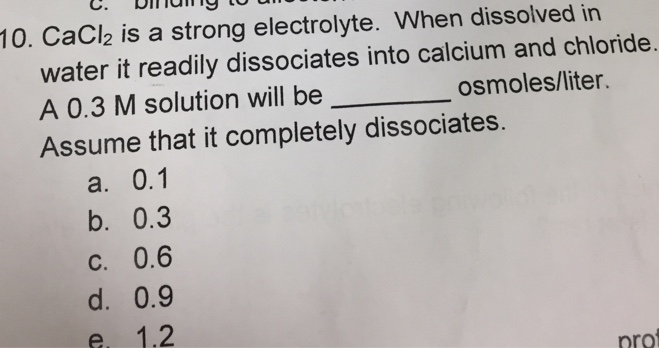# Problem: CaCl2 is a strong electrolyte. When dissolved in water, it readily dissociates into calcium and chloride. A 0.3 M solution will be _______ osmoles/liter.Assume that it completely dissolves.A. 0.1B. 0.3C. 0.6D. 0.9E. 1.2

###### FREE Expert Solution

It is assumed that the dissolution is complete. And for this sample, we have a solution of CaCl2, which dissociates into three particles per unit of substance, one Ca2+ and two Cl-.###### Problem Details

CaCl2 is a strong electrolyte. When dissolved in water, it readily dissociates into calcium and chloride. A 0.3 M solution will be _______ osmoles/liter.

Assume that it completely dissolves.

A. 0.1

B. 0.3

C. 0.6

D. 0.9

E. 1.2